NIUHE

Deep NLP - Conditional Language Model

A conditional language model assigns probabilities to sequences of words, $w = (w_1, w_2, …, w_l)$, given some conditioning context, x.

As with unconditional models, it is again helpful to use the chain rule to decompose this probability: $p(w|x) = \prod_{t=1}^lp(w_t|x, w_1, w_2, ..., w_{t-1})$

x "input" w "text output"
An author A document written by that author
A topic label An article about that topic
{SPAM, NOT_SPAM} An email
A sentence in French Its English translation
A sentence in English Its French translation
A sentence in English Its Chinese translation
An image A text description of the image
A question + a document Its answer
A question + an image Its answer

To train contitional language models, we need paired samples, $\{(x_i, w_i)\}$.

Algorighmic challenges

We often want to find the most likely $w$ given some $x$. This is unfortunately generally and intractable problem. $w^* = \arg \max_wp(w|x)$ We therefore approximate it using a beam search or with Monte Carlo methods since $w^{(i)} \approx p(w|x)$ is often computationally easy.

Evaluating conditional LMs

We can use cross entropy or preplexity, it's okay to implement, but hard to interpret.

Task-specific evaluation. Compare the model's most likely output to human-generated expected output using a task-specific evaluation metric $L$. $w^* = \arg \max_wp(w|x)\ \ \ \ \ L(w^*, w_{ref})$ Examples of $L$: BLUE, METEOR, WER, ROUGE, easy to implement, okay to interpret.

Encoder-Decoder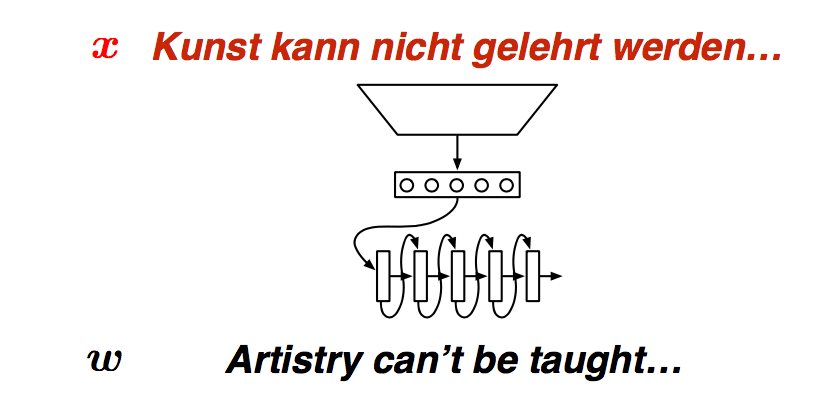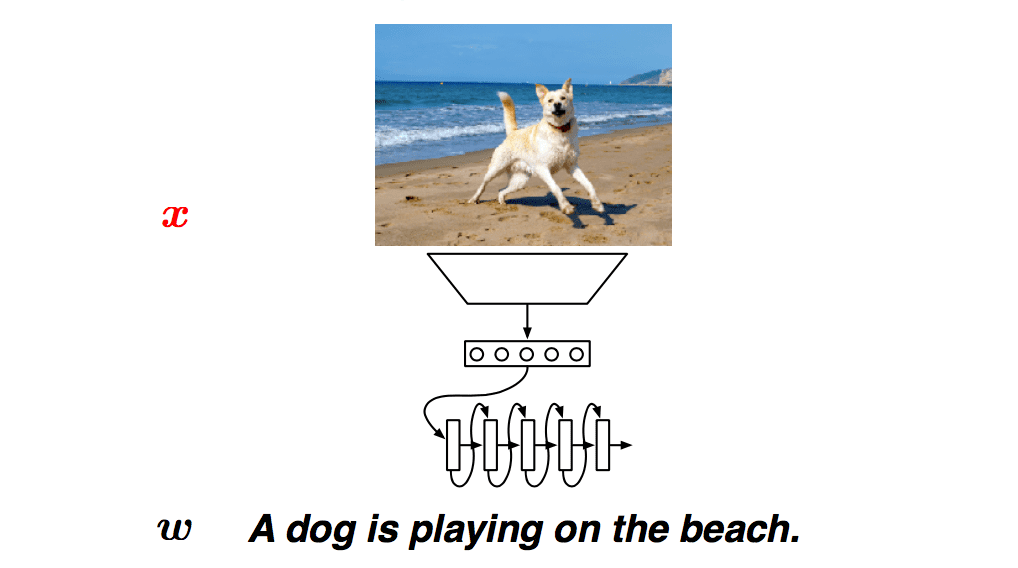Two questions

• How do we encode $x$ as a fixed-size vector, $c$ ?
• How do we condition on $c$ in the decoing model?

Kalchbrenner and Blunsom 2013

Encoder $c = embed(x)$

$s = Vc$

Recurrent decoder $h_t = g(W[h_{t-1}; w_{t-1}] + s + b)$

$u_t = Ph_t + b'$

$p(W_t|x, w<t) = softmax(u_t)$

CSM Encoder

How should we define $c = embed(x)$ ?

Convolutional sentence model(CSM)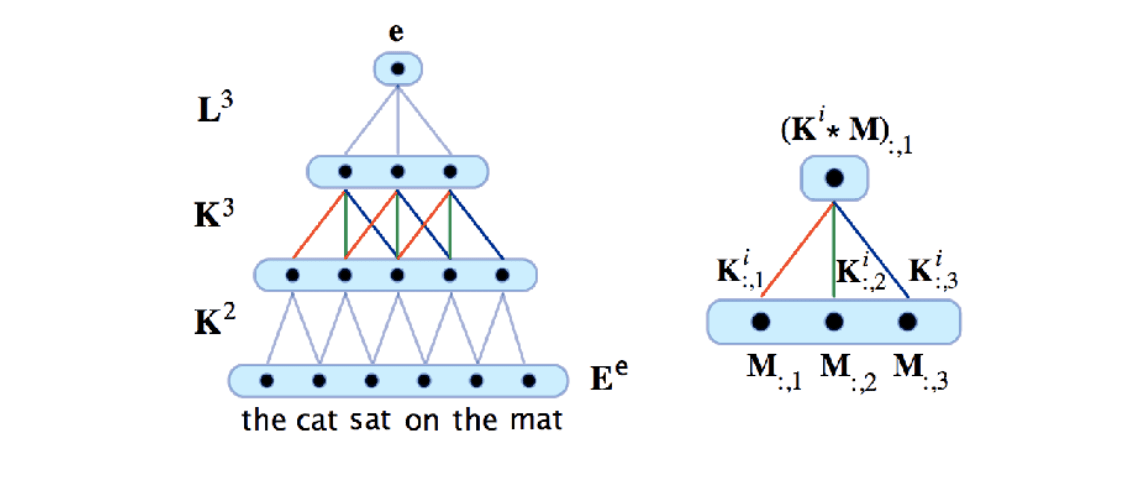Good

• By stacking them, longer range dependencies can be learnt
• Convolutions learn interactions among features in a local context
• Deep ConvNets have a branching structure similar to trees, but no parser is required

• Sentences have different lengths, need different depth trees; convnets are not usually so dynamic, but see Kalchbrenner et al. (2014). A convolutional neural network for modelling sentences. In Proc. ACL.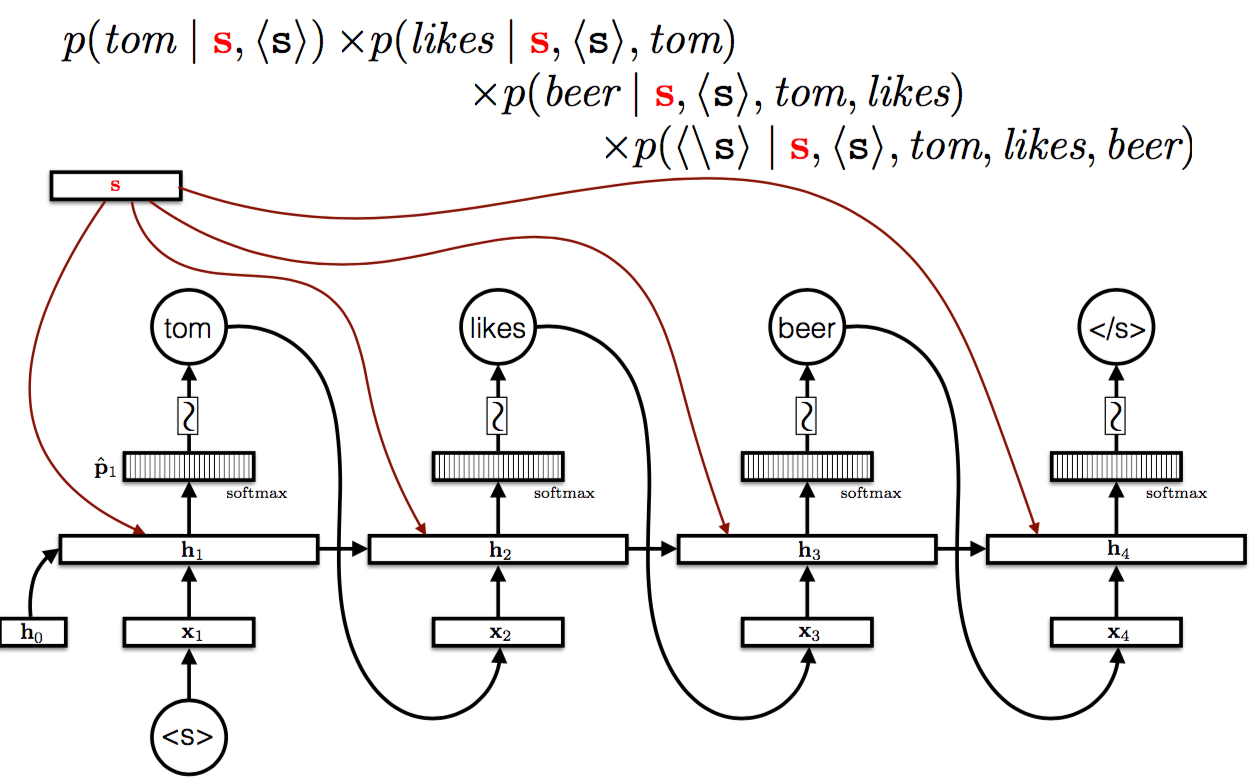Sutskever et al. (2014)

LSTM encoder

• $(c_0, h_0)$ are parameters
• $(c_i, h_i)$ = LSTM($x_i, c_{i-1}, h_{i-1}$)

The encoding is $(c_l, h_l)$ where $l = |x|$

LSTM decoder

• $w_0 = <s>$
• $(c_{t+l}, h_{t+l}) = LSTM(w_{t-1}, c_{t+l-1}, h_{t+l-1})$
• $u_t = Ph_{t+l} + b$
• $P(W_t|x, w<t) = softmax(u_t)$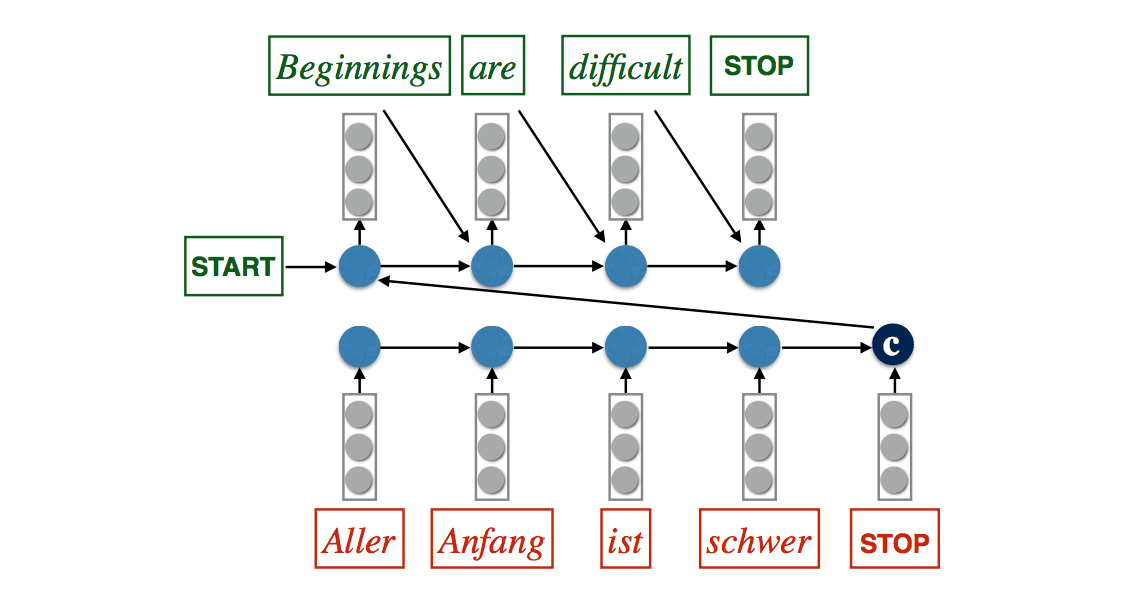Good

• RNNs deal naturally with sequences of various lengths
• LSTMs in principle can propagate gradients a long
• Very simple architecture

• The hidden state has to remember a lot of information!

Tricks

Read the input sequence "backwards" : +4 BLEU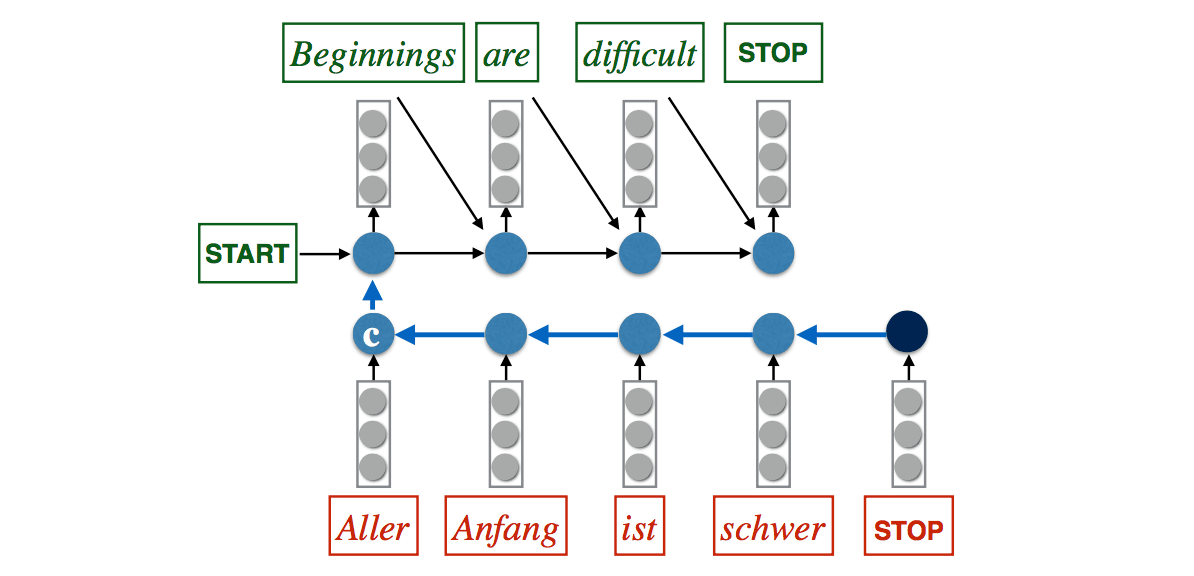Use an ensemble of J independently trained models.

• Ensemble of 2 models: +3 BLEU
• Ensemble of 5 models; +4.5 BLEU

In general, we want to find the most probable (MAP) output given the input, i.e. \begin{align} w^* = \arg\max_{w}p(w|x) = \arg \max_w\sum_{t=1}^{|w|}\log p(w_t|x, w_{<t}) \end{align} This is, for general RNNs, a hard problem. We therefore approximate it with a greedy search$\begin{array}{lcl} w_1^* = \arg\max_{w_1}p(w_1|x)\\ w_2^* = \arg\max_{w_2}p(w_2|x, w_1^*)\\ ...\\ w^*_t = \arg\max_{w_t}p(w_t|x, w^*_{<t}) \end{array}$ A slightly better approximation is to use a beam search with beam size $b$. Key idea: keep track of top b hypothesis. Use beam search: +1 BLEU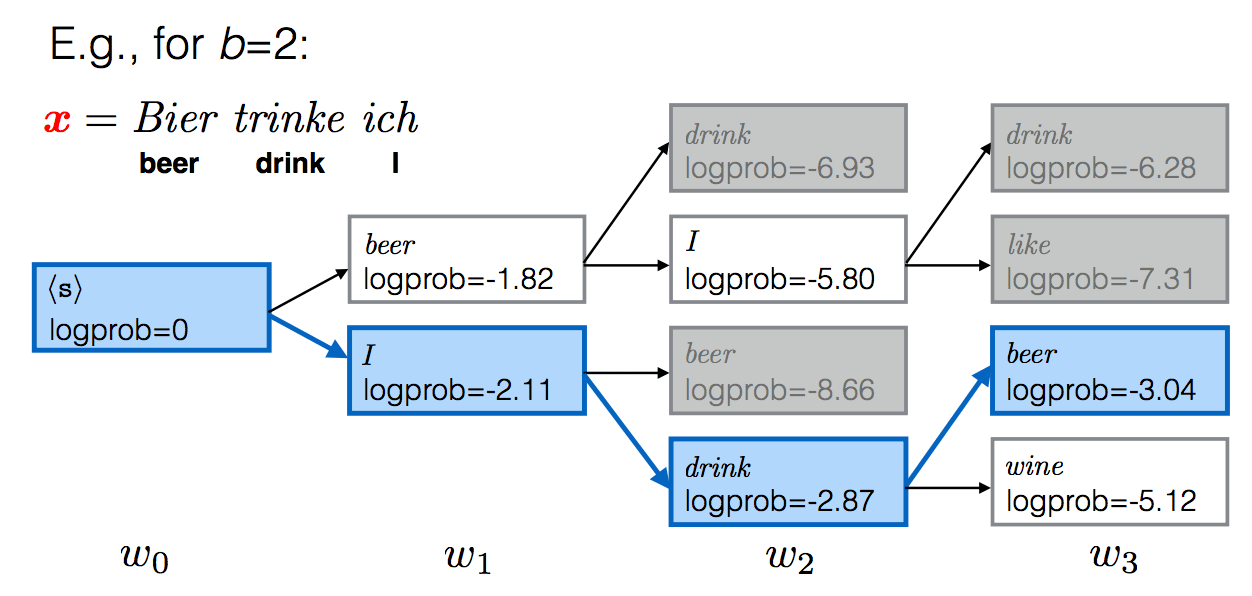Kiros et al.(2013)

Image caption generation

• Neural networks are great for working with multiple modalities - Everything is a vector!
• Image caption generation can therefore use the same techniques as translation modeling
• Relatively few captioned images are avaliable
• Pre-train image embedding model using another task, like image identification (e.g., ImageNet)

Look a lot like Kalchbrenner and Blunsom(2013)

• convolutional network on the input
• n-gram language model on the output

Innovation: multiplicative interactions in the decoder n-gram model

Encoder x = enbed($x$)

Simple conditional n-gram LM: $\begin{array}{lcl} h_t = W[w_{t-n+1}; w_{t-n+2};...; w_{t-1}] + Cx\\ u_t = Ph_t+b\\ p(W_t|x, w_{t-n+1}^{t-1}) = softmax(u_t) \end{array}$ Multiplicative n-gram LM:

• $w_i = r_{i,j,w}x_j$
• $w_i = u_{w,i}v_{i,j}\ \ \ \ \ \ \ \ (U\in R^{|V|*d}, V \in R^{d*k})$
• $r_t = W[w_{t-n+1}; w_{t-n+2};...; w_{t-1}] + Cx$
• $h_t = (W^{fr}r_t)\odot (W^{fx}x)$
• $u_t = Ph_t + b$
• $p(W_t|x, w_{<t}) = softmax(u_t)$

Two messages:

• Feed-forward n-gram models can be used in place of RNNs in conditional models
• Modeling interactions between input modalities holds a lot of promise
• Although MLP-type models can approximate higher order tensors, multiplicative models appear to make learning interactions easier